Mathematical Formulas for Prion All Cross-Structures Listed in the Protein Data Bank | OMICS International
Medicinal Chemistry

Like us on:

All submissions of the EM system will be redirected to Online Manuscript Submission System. Authors are requested to submit articles directly to Online Manuscript Submission System of respective journal.

# Mathematical Formulas for Prion All Cross-Structures Listed in the Protein Data Bank

Jiapu Zhang1,2*

1Molecular Model Discovery Laboratory, Department of Chemistry and Biotechnology, Faculty of Science, Engineering and Technology, Swinburne University of Technology, Hawthorn Campus, Hawthorn, Victoria 3122, Australia

2Graduate School of Sciences, Information Technology and Engineering and Centre of Informatics and Applied Optimisation, Faculty of Science and Technology, The Federation University Australia, Mount Helen Campus, Mount Helen, Ballarat, Victoria 3353, Australia

*Corresponding Author:
Jiapu Zhang
Molecular Model Discovery Laboratory
Department of Chemistry and Biotechnology
Faculty of Science Engineering and Technology
Swinburne University of Technology Hawthorn Campus
Hawthorn, Victoria 3122, Australia
Tel:
+61-3-92145596/+61-3-53276335
E-mail: [email protected]/[email protected]

Received date: February 20, 2016; Accepted date: March 29, 2016; Published date: March 31, 2016

Citation: Zhang J (2016) Mathematical Formulas for Prion All Cross-Structures Listed in the Protein Data Bank. Med chem (Los Angeles) 6:179-188. doi:10.4172/2161-0444.1000343

Copyright: © 2016 Zhang J. This is an open-access article distributed under the terms of the Creative Commons Attribution License, which permits unrestricted use, distribution, and reproduction in any medium, provided the original author and source are credited.

Visit for more related articles at Medicinal Chemistry

#### Abstract

Prion protein (PrP) has two regions: unstructured region PrP(1-120) and structured region PrP(119-231). In the structured region, there are many segments which have the property of amyloid fibril formation. By theoretical calculations, PrP(126-133), PrP(137-143), PrP(170-175), PrP(177-182), PrP(211-216) have the amyloid fibril forming property. PrP(142-166) has a X-ray crystallography experimental β-hairpin structure, instead of a pure cross-β amyloid fibril structure; thus we cannot clearly find it by our theoretical calculations. However, we can predict that there must be a laboratory X-ray crystal structure in PrP(184-192) segment that will be produced in the near future. The experiments of X-ray crystallography laboratories are agreeing with our theoretical calculations. This article summarized mathematical formulas of prion amyloid fibril cross-β structures of all the above PrP segments currently listed in the Protein Data Bank.

#### Keywords

PrP structured region; Amyloid fibril formation peptides; Theoretical calculations; Experimental laboratories; Mathematical formulas

#### Introduction

Table 1 lists the cross-β structures of all PrP segments that were listed in the Protein Data Bank (PDB, www.rcsb.org), produced by X-ray crystallography experiments. In the below, 1- 24 give some mathematical formulas to describe all these cross-β structures.

 PrP segment Species PDB ID Class of the cross-β 1  PrP(126–131) human 4TUT Class 7[1,4] 2 human 4UBY Class 8[1,4] 3 human 4UBZ Class 8[1,4] 4  PrP(126–132) human 4W5L Class 8[1,4] 5 human 4W5M Class 8[1,4] 6 human 4W5P Class 8[1,4] 7  PrP(127–133) human 4W5Y Class 6[1,4] 8 human 4W67 Class 6[1,4] 9 human 4W71 Class 6[1,4] 10  PrP(127–132) human 4WBU Class 8[1,4] 11 human 4WBV Class 8[1,4] 12 human 3MD4 antiparallel (P 21 21 21 ) 13 human 3MD5 parallel (P 1 21 1) 14 human-M129 3NHC Class 8 15 human-V129 3NHD Class 8 16  PrP(137–142) mouse 3NVG Class 1 17  PrP(137–143) mouse 3NVH Class 1 18  PrP(138–143) Syrian hamster 3NVE Class 6 19 human 3NVF Class 1 20  PrP(142–166) sheep 1G04 β-hairpin 21  PrP(170–175) human 2OL9 Class 2 22 elk 3FVA Class 1 23  PrP(177–182, 211–216) human 4E1I β-prism I fold (P 21 21 21 ) 24 human 4E1H β-prism I fold(P 21 21 21 )

Table 1: The cross-β structures known in the PDB Bank of PrP segments.

1. Figure 1, the mathematical formula for B Chain got from A Chain is

Figure 1: Protein fibril structure of human M129 prion GGYMLG (126–131) (PDB ID: 4TUT). The dashed lines denote the hydrogen bonds. A, B, C, D denote the chains of the fibril.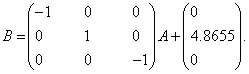(1)

2. Figure 2, mathematical formulas for EFGH, IJKL, MNOP Chains obtained from ABCD Chains respectively are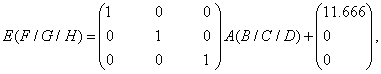(2)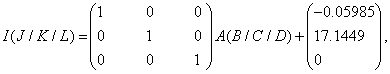(3)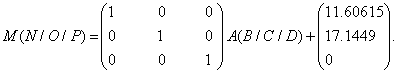(4)

Figure 2: Protein fibril structure of human V129 prion GGYVLG (126–131) (PDB ID: 4UBY). The dashed lines denote the hydrogen bonds. A, B, C, D denote the chains of the fibril.

3. Figure 3, mathematical formulas for CD, EF, GH, IJ, KL Chains obtained from AB Chains respectively are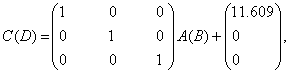(5)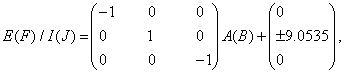(6)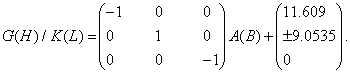(7)

Figure 3: Protein fibril structure of human L129 prion GGYLLG (126–131) (PDB ID: 4UBZ). The dashed lines denote the hydrogen bonds. A, B denote the basic chains of the fibril.

4. Figure 4, mathematical formulas for CD, EF, GH, IJ, KL Chains obtained from the basic AB Chains respectively are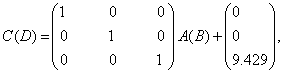(8)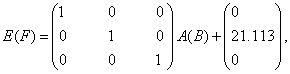(9)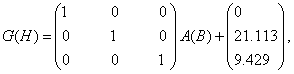(10)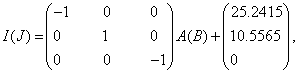(11)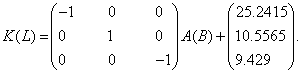(12)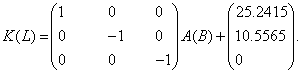(13)

Figure 4: Protein fibril structure of human L129 prion GGYLLGS (126–132) (PDB ID: 4W5L). The dashed lines denote the hydrogen bonds. A, B denote the basic chains of the fibril.

5. Figure 5, mathematical formulas for CD, EF, GH, IJ Chains obtained from the basic AB Chains respectively are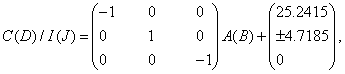(14)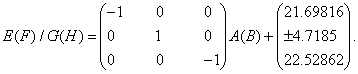(15)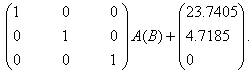(16)

Figure 5: Protein fibril structure of human M129 prion GGYMLGS (126–132) (PDB ID: 4W5M). The dashed lines denote the hydrogen bonds. A, B denote the basic chains of the fibril.

6. Figure 6, mathematical formulas for CD, EF, GH Chains obtained from the basic AB Chains respectively are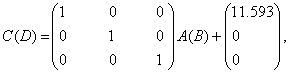(17)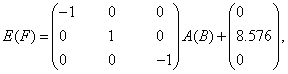(18)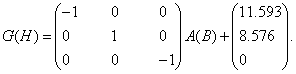(19)

Figure 6: Protein fibril structure of human V129 prion GGYVLGS (126–132) (PDB ID: 4W5P). The dashed lines denote the hydrogen bonds. A, B, C, D, E, F, G, H denote the chains of the fibril.

7. Figure 7, mathematical formulas for CD, EF, G H Chains obtained from the basic AB Chains respectively are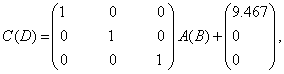(20)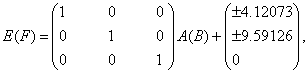(21)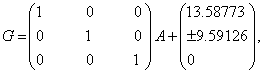(22)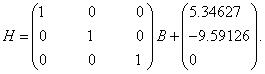(23)

Figure 7: Protein fibril structure of human M129 prion GYMLGSA (127–133) (PDB ID: 4W5Y). The dashed lines denote the hydrogen bonds. A, B denote the basic chains of the fibril.

8. Figure 8, mathematical formulas for CD, EF, GH Chains obtained from the basic AB Chains respectively are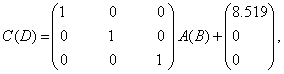(24)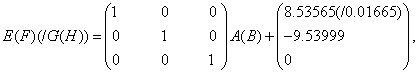(25)

Figure 8: Protein fibril structure of human V129 prion GYVLGSA (127–133) (PDB ID: 4W67). The dashed lines denote the hydrogen bonds. A, B denote the basic chains of the fibril.

9. Figure 9, mathematical formulas for CD, EF, GH Chains obtained from the basic AB Chains respectively are(26)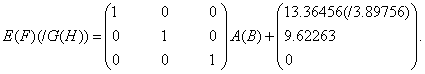(27)

Figure 9: Protein fibril structure of human L129 prion GYLLGSA (127–133) (PDB ID: 4W71). The dashed lines denote the hydrogen bonds. A, B denote the basic chains of the fibril.

10. Figure 10, mathematical formulas for CD, EF, GH Chains obtained from the basic AB Chains respectively are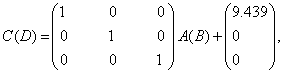(28)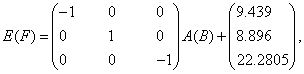(29)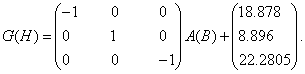(30)

Figure 10: Protein fibril structure of human M129 prion GYMLGS (127–132) (PDB ID: 4WBU). The dashed lines denote the hydrogen bonds. A, B denote the basic chains of the fibril.

11. Figure 11, mathematical formulas for CD, EF, GH Chains obtained from the basic AB Chains respectively are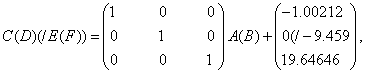(31)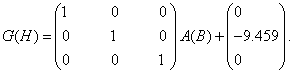(32)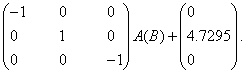(33)

Figure 11: Protein fibril structure of human V129 prion GYVLGS (127–132) (PDB ID: 4WBV). A, B denote the basic chains of the fibril.

12. Figure 12, basing on AB Chains, other chains CD, EF, GH in the asym-metric one unit cell can be obtained by the mathematical formulas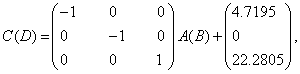(34)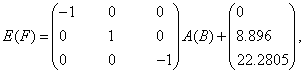(35)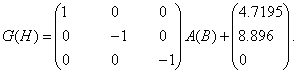(36)

Figure 12: Protein fibril structure of human M129 prion GYMLGS (127–132) (PDB ID: 3MD4). The dashed lines denote the hydrogen bonds. A, B, C, D, E, F, G, H denote the chains of the fibril.

13. Figure 13, basing on AB Chains, other Chains CD in the asymmetric unit cell can be obtained by mathematical formula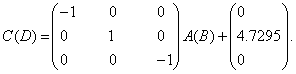(37)

Figure 13: Protein fibril structure of human V129 prion GYMLGS (127–132) (PDB ID: 3MD5). The dashed lines denote the hydrogen bonds. A, B, C, D denote the chains of the fibril.

14. Figure 14 we see that G(H) chains (i.e., β-sheet 2) of 3NHC.pdb can be obtained from A(B) chains (i.e., β-sheet 1) by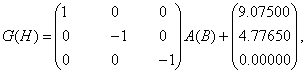(38)

Figure 14: Protein fibril structure of human M129 prion GYMLGS (127–132) (PDB ID: 3NHC). The dashed lines denote the hydrogen bonds. A, B ... K, L denote the chains of the fibril.

and other chains can be got by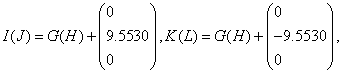(39)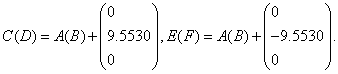(40)

15. By observations of the 3rd column of coordinates of 3NHD.pdb and Figure 15, G(H) chains (i.e., β-sheet 2) of 3NHD.pdb can be calculated from A(B) chains (i.e., β-sheet 1) by Equation (41) and other chains can be calculated by Equations (42)~(43):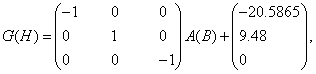(41)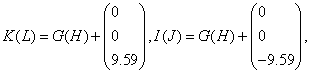(42)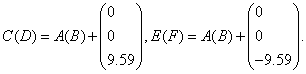(43)

Figure 15: Protein fibril structure of human V129 prion GYVLGS (127-132) (PDB ID: 3NHD). The dashed lines denote the hydrogen bonds. A, B ... K, L denote the chains of the fibril.

16. In Figure 16 we see that H Chain (i.e., β-sheet 2) of 3NVG.pdb can be obtained from A Chain (i.e., β-sheet 1) by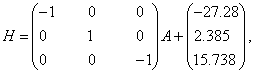(44)

Figure 16: Protein fibril structure of MIHFGN segment 137–142 from mouse prion (PDB ID: 3NVG). The purple dashed lines denote the hydrogen bonds. A, B, ..., I, J denote the 10 chains of the fibril.

and other chains can be got by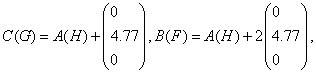(45)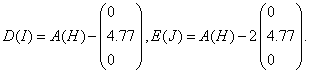(46)

17. In Figure 17 we see that H chain (i.e., β-sheet 2) of 3NVH.pdb can be obtained from A chain (i.e., β-sheet 1) by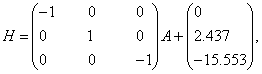(47)

Figure 17: Protein fibril structure of MIHFGND segment 137–143 from mouse prion (PDB ID: 3NVH). The purple dashed lines denote the hydrogen bonds. A, B, ..., I, J denote the 10 chains of the fibril.

and other chains can be got by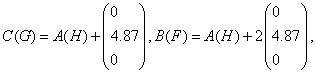(48)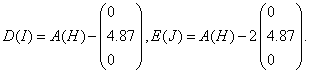(49)

18. In Figure 18 we see that G(H) chains (i.e., β-sheet 2) of 3NVE.pdb can be obtained from A(B) chains (i.e., β-sheet 1) by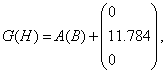(50)

Figure 18: Protein fibril structure of MMHFGN segment 138–143 from Syrian Hamster prion (PDB ID: 3NVE). The purple dashed lines denote the hydrogen bonds. A, B, ..., K, L denote the 12 chains of the fibril.

and other chains can be got by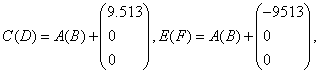(51)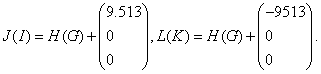(52)

19. In Figure 19 we see that H chain (i.e., β-sheet 2) of 3NVF.pdb can be obtained from A chain (i.e., β-sheet 1) by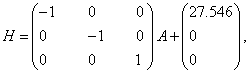(53)

Figure 19: Protein fibril structure of IIHFGS segment 138–143 from human prion (PDB ID: 3NVF). The purple dashed lines denote the hydrogen bonds. A, B, ..., I, J denote the 10 chains of the fibrils.

and other chains can be got by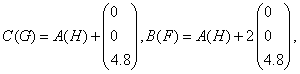(54)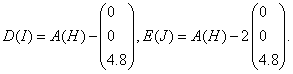(55)

20. Figure 20.

Figure 20: Protein β-hairpin structure of segment 142–166 from sheep prion (PDB ID: 1G04). The dashed lines denote the hydrogen bonds.

21. In Figure 21, we see that the D chain (i.e., β-sheet 2) of 2OL9.pdb can be obtained from A Chain (i.e., β-sheet 1) using the mathematical formula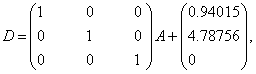(56)

Figure 21: Protein fibril structure of NNQNTF segment 170–175 from human prion (PDB ID: 2OL9). The dashed lines denote the hydrogen bonds. A, B, C, D, E, F denote the 6 chains of the fibrils.

and other chains can be got by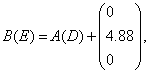(57)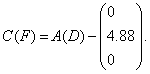(58)

22. In Figure 22, we see that the D chain (i.e., β-sheet 2) of 3FVA. pdb can be obtained from A Chain (i.e., β-sheet 1) using the mathematical formula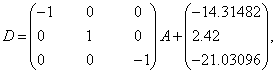(59)

Figure 22: Protein fibril structure of NNQNTF segment 170–175 from elk prion (PDB ID: 3FVA). The dashed lines denote the hydrogen bonds. A, B, C, D, E, F denote the 6 chains of the fibrils.

and other chains can be got by(60)(61)

23. Figure 23.

Figure 23: Protein β-prism fibril structure of segments 177–182, 211–216 from human prion (PDB ID: 4E1I). The dashed lines denote the hydrogen bonds. A, B, C, D, E, F, G, H, I, J, K, L denote the 12 chains of the fibrils.

24. Figure 24.

Figure 24: Protein β-prism fibril structure of segments 177–182, 211–216 from human prion (PDB ID: 4E1H). The dashed lines denote the hydrogen bonds. A, B, C, D, E, F, G, H, I, J, K, L denote the 12 chains of the fibrils.

All the above X-ray crystallography structures show to us, in the PrP(119-231) structured region, there are many segments of peptides which can formulate into amyloid fibrils. The author’s theoretical calculations [5,6] (Figures 25 and 26, where -26 kcal/mol is the threshold energy of amyloid fibril formation: if energy is less than -26 kcal/mol it will have amyloid fibril forming property ) also show this point.

Figure 25: By theoretical calculations of the author, PrP(126-133), PrP(137-143), PrP(170-175), PrP(177-182), PrP(211-216) were identified owning the amyloid fibril forming property respectively (the graphs from left to right).

Figure 26: To identify the segments owning the amyloid fibril forming property in the whole PrP structured region: if the energy is less than -26 kcal/mol it will have amyloid fibril forming property .

Last, seeing Figure 26, we may think there must be a laboratory X-ray crystal β-type structure in PrP(184-192) segment that will be produced by a X-ray lab in the near future.

In conclusion, this short article described about the comparison study of theoretical calculations and X-ray crystallography study of prion protein cross-beta structures. The author has very good prediction of theoretical calculations using mathematical formulas of unstructured region and structured region of prion proteins in detail well demonstrated. Moreover, the X-ray crystallography structures showed, in the PrP(119–231) structured region, there are many segments of peptides which can formulate into amyloid fibrils. The author’s theoretical calculations for Figures 25 and 26 were shown threshold energy as -26 kcal/mol, which supports to the amyloid fibril formation. Even if the threshold energy is less than -26 kcal/mol also they will have amyloid fibril forming property. This is a good piece of work useful for the medicinal chemists. The author presented the results very well in the article, and provided an introduction of the structures to understand the significance in the biological use of amyloid fibrils [8-14].

#### Acknowledgements

This research was supported by a Victorian Life Sciences Computation Initiative (VLSCI) grant numbered FED0001 on its Peak Computing Facility at the University of Melbourne, an initiative of the Victorian Government (Australia). The author also acknowledges the anonymous referees for their numerous insightful comments and references [8-14] offered.

#### References

Select your language of interest to view the total content in your interested language

### Article Usage

• Total views: 8717
• [From(publication date):
March-2016 - Nov 22, 2019]
• Breakdown by view type
• HTML page views : 8620

## Review summary

1. Fitin
Posted on Sep 29 2016 at 8:00 pm
This is a good piece of work useful for the medicinal chemists. Authors have very good prediction of theoretical calculations using mathematical formulas of unstructured region and structured region of prion proteins in detail well demonstrated.Can't read the image? click here to refresh